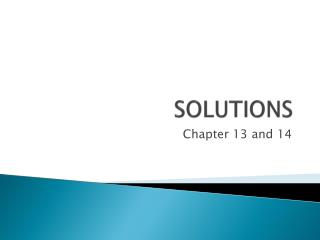Download PresentationSOLUTIONS

# SOLUTIONS

Download Presentation## SOLUTIONS

- - - - - - - - - - - - - - - - - - - - - - - - - - - E N D - - - - - - - - - - - - - - - - - - - - - - - - - - -
##### Presentation Transcript

1. SOLUTIONS Chapter 13 and 14

2. VOCABULARY • Solution • Solute • Solvent • Soluble • Solubility • Solution equilibrium • Saturated solution • Supersaturated solution • Solvation (hydration) • Electrolyte

3. The Solution Process • Factors affecting rate of dissolution 1. Increasing solute surface area • Increase by crushing the solute • Exposes more solute to solvent and dissolution occurs more rapidly 2. Agitation • Helps disperse the solute particles and bring fresh solvent to the surface of the solute 3. Heating a solvent • Increases the kinetic energy of the solvent particles causing more collisions with the solute

4. SOLUBILITY • Defined as: the amount of a substance required to form a saturated solution with a specific amount of solvent at a specified temperature. • Usually reported as g/ 100 g solvent • Ex. Sugar 204 grams per 100 g of water at 20ºC.

5. What affects solubility?POLARITY • General rule: “likes dissolve likes” • Non-polar solvents dissolve non-polar solutes • Miscible Benzene CCl4 C6H5CH3 (tolulene) • Polar solvents dissolve polar and ionic solutes H2O and NaCl • C2H5OH – ethanol (slightly polar) • will NOT dissolve ionic compounds

6. TEMPERATURE affects solubility of solids

7. Gas solubility • Henry’s law – gas solubility increases as the partial PRESSURE of the gas above the solvent increases. • Gases become less soluble as temperature increases.

8. CONCENTRATION of SOLUTIONS • A measure of the amount of solute in a given amount of solvent or solution. Can be expressed using: • Molarity • molality • Mass % • Parts per million (ppm) • Parts per billion (ppb)

9. MOLARITY • Number of moles of solute/ Liters of solution. • Symbolized: M • M = moles solute/ L soln. • Ex. 6.0 M HCl

10. MOLARITY 1. What is the molarity of a solution prepared by dissolving 150.0 grams of sodium carbonate in enough water to make 1.0 L of solution. 2. Given 35.0 ml of a 0.50 M solution of HCl, how many moles of HCl are present?

11. examples 3. How many grams of NaCl are present in a 3.0 M solution? 4. What volume of 3.00 M H2SO4 is needed for a reaction that requires 146.3 grams of sulfuric acid?

12. molality • The concentrations of a solution expressed in moles of solute per kilogram of solvent. • Used for freezing point and boiling point problems.

13. examples • Ex. What is the molality of a solution prepared by mixing 15 grams of glucose into 500 grams of water? • What is the molality of a salt solution made by adding 5.0 kg of calcium chloride to 200 kg of ice?

14. Colligative properties • What are the effects of adding a solute to a solvent? • Colligative properties – depend on the number of particles in solution, not the type of particles. • How many particles are present when the following dissolve? • NaCl • C6H12O6 • CaCl2

15. Boiling point elevation • Boiling point – • The temperature at which the vapor pressure of the liquid = the atmospheric pressure • The boiling point of a solvent will increase when a non-volatile solute is added. • Why? Particles block the surface of the solution, making it harder for the solvent (g) to escape. More ke is required, so the temperature must increase. • How much? ∆Tb = i Kb m Where i = number of particles Kb = boiling point elevation constant (+.51°C/molal for water) m = molality

16. examples • What is the boiling point elevation when 150.0 grams of sucrose is added to 850. grams of water? • What is the boiling point elevation when 0.50 grams of sodium chloride is added to 2.0 kg of water?

17. Freezing point depression • Freezing point – the temperature at which a solution forms a solid from the (aq) phase • The freezing point of a solvent will decrease when a non-volatile solute is added. • Why? • Solute particles interfere with the organization of the solid. In order to form the solid around the solute particles, the kinetic energy must decrease, so the freezing point is lowered. • How much? ∆Tf = iKfm Where i = number of particles Kf = freezing point depression constant (-1.86°C/molal for water) m = molality

18. examples What is the freezing point depression when 500. grams of sodium chloride is added to 2000. g of water? What is the freezing point depression when 50.0 grams of calcium chloride is added to 500. grams of water?

19. STOICHIOMETRY • How many ml of 3.0 M NaOH are needed to neutralize 5.0 grams of acetic acid found in a vinegar solution? What is the M of the vinegar? • How many grams of Mg can be dissolved by 15.0 ml of 6.0 M HCl? How much gas will be produced by this reaction at STP?

20. STOICHIOMETRY • 5.0 ml of 6.0 M HCl reacts with 25.0 ml .10 M NaOH. How much water will be produced? • How many ml 0.10 M NaOH should be used to neutralize the 5.0 ml of HCl?